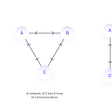# 1. Sum of a 2D matrix with least amount of code

`adjacencyList = [[1, 0, 0], [1, 1, 0], [0, 1, 1]]totalSum = sum(sum(adjacency, []))print(totalSum)> 5`
`adjacencyList = [[1, 0, 0], [1, 1, 0], [0, 1, 1]]partialStep = sum(adjacencyList, [])print(partialStep)> [1, 0, 0, 1, 1, 0, 0, 1, 1]`
`a = [3, 2]b = [4, 5]c = a + b`

# 2. Creation of a 2D matrix succinctly

`array2D = [[0 for _ in range(rows)] for _ in range(cols)]`

# 3. Adding on a list as we traverse it

`initial = [1, 2, 3, 4]others = [5, 6, 7]result = []for element in initial:  result.append(element)  if others:    initial.append(others.pop(0))print(result)> [1,2,3,4,5,6,7]`
`initial = [1, 2, 3, 4]others = [5, 6, 7]result = []for i in range(len(initial)):  result.append(initial[i])  if others:    initial.append(others.pop(0))print(result)print(result)> [1,2,3,4]`

# 4. Using DefaultDict and not Dictionary

`aDict = dict()for i in range(10):  for j in range(10):    if j == 0:      aDict[i] = [] #set-up of a list using an if-condition        aDict[i].append(j)`
`from collections import defaultdict`
`aDictOfList = defaultdict(list) # notice the use of the list class for i in range(10):  for j in range(10):    aDictOfList[i].append(j) # we just append to the list print(aDictOfList)`
`from collections import defaultdictaDictOfZeros = defaultdict(int) # int as parameterfor i in range(3):  print(aDictOfZeros[i])print(aDictOfZeros)> 000`

# 5. Initializing a DefaultDict that contains Non-Zeros

`from collections import defaultdictfourDict = defaultdict(lambda: 4)result = []for i in range(10):  result.append(fourDict[i])print(result)`

# 6. Using Decorator to time function calls

`def aFunction():  def nested_function():    return "I am nested"  result = f"I called nested function and the output is {nested_function()}"  return result> aFunction() `
`import timedef profile_time(function): # name of decorator function  def wrapper_function(): # always have nested "wrapper_function"    before = time.time()    function()    after = time.time()    profile_result = f"time taken for {function.__name__} is {after - before}"    return profile_result  return wrapper_function # return the wrapper function - no ()"@profile_timedef simple_loop():  for i in range(1000000):     i += 10> profile_time() # will run function and show the time taken `

`import syssys.setrecursionlimit(10**6)`

--

--

--

## More from Mipsmonsta

Writing to soothe the soul, programming to achieve flow

Love podcasts or audiobooks? Learn on the go with our new app.

## Let Siri Control Your Raspberry## Creating Modular Waypoint System for Enemy AI in Unity 2021## The road to openpilot 1.0## The network effects of HUMAN Protocol## C# Quicktip: In Xunit how to skip a unit test from being run## Fascinating Data Structure: The Hash Table## Interesting Electronic Components #1: HLS-LFCD2## Understanding The Memcached Source Code — Event Driven I## Mipsmonsta

Writing to soothe the soul, programming to achieve flow

## Things You Didn’t Know About Python Assertion## This Python Coding Interview Question Gave Me PTSD## Where does Python “go”?## How to Quicksort in Python Using List Comprehension